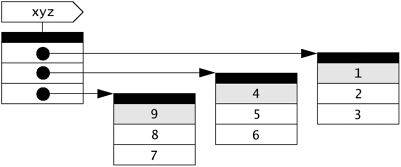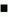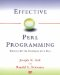# Item 35: Use map and grep to manipulate complex data structures.

### Item 35: Use map and grep to manipulate complex data structures.

Sometimes it's useful to take a "slice" out of a multidimensional array or hash, or to select slices that have certain characteristics. Conversely, you may need to assemble a collection of lists into a 2-D array, or perhaps assemble a collection of 2-D arrays into a 3-D array. Perl's map and grep operators are a perfect choice for chores like these.

#### Slicing with map

Let's begin with a program that reads a file containing 3-D coordinates into memory:

Reading a file of 3-D coordinates into memory

 This program will read a file of 3-D coordinates into memory. Each line of the file will contain the x, y, and z coordinates of a single point, separated by whitespace. For example: ` # point data 1 2 3 4 5 6 9 8 7 ` ` open POINTS, "points" or die "couldn't read points data: \$!\n"; while () { next if /^\s*#.*\$/; push @xyz, [ split ]; } ` Skip comments, then split a line into 3 values, put 'em in an anonymous array, and append it to @xyz . ` foreach \$pt (@xyz) { print "point ", \$i++, ": x = \$pt->, y = \$pt->, ", "z = \$pt->\n"; } ` Prints: point 1: x = 1, y = 2, z = 3 point 2: x = 4, y = 5, z = 6 point 3: x = 9, y = 8, z = 7

The point data is read into a structure that looks like the following:Now, let's suppose you would like to have just the x (0th) element from each point, as indicated by the shading in the figure. You could write a loop using an explicit index, or perhaps use a foreach loop:

 ` for (\$i = 0; \$i < @xyz; \$i++) { push @x, \$xyz[\$i]; } ` Here's a for loop with an explicit index. ` foreach (@xyz) { push @x, \$_->; } ` The same general idea, with a foreach loop.

But, really, this is a natural application for map :Use map to take slices of complex data structures.

 ` @x = map { \$_-> } @xyz; ` Select the 0th element from each anonymous array in @xyz .

#### Nesting with map

On the other hand, suppose that you are starting out with parallel arrays @x , @y , and @z containing vectors of points:You now would like to assemble them into a single 3-D structure like the one shown earlier. Once again, you could use some sort of explicit looping structure:

 ` for (\$i = 0; \$i < @x; \$i++) { \$xyz[\$i] = \$x[\$i]; \$xyz[\$i] = \$y[\$i]; \$xyz[\$i] = \$z[\$i]; } ` Turn @x , @y , and @z into @xyz , the slow and tedious way.

However, map provides a much more elegant alternative:Use [ ] inside map to create more deeply nested structures.

 ` @xyz = map { [ \$x[\$_], \$y[\$_], \$z[\$_] ] } 0 .. \$#x; ` Turn @x , @y , and @z into @xyz , the Perl-ish way.

No doubt you can envision a host of variations on the slicing and nesting themes. For example, switching the x (0th) and y (1st) coordinates:

 ` @yxz = map { [ \$_->, \$_->, \$_-> ] } @xyz; ` Swap x and y coordinates. ` @yxz = map { [ @\$_[1, 0, 2] ] } @xyz; ` It's prettier using a slice.

Or, perhaps, creating a new list containing the magnitudes of the points:

 ` @mag = map { sqrt( \$_-> * \$_-> + \$_-> * \$_-> + \$_-> * \$_-> ) } @xyz; ` Compute magnitude of each point in @xyz and put results into a list.

The Schwartzian Transform (see Item 14) is an application that uses both slicing and nesting operations with map :

 ` @sorted_by_mtime = map { \$_-> } sort { \$a-> <=> \$b-> } map { [ \$_, -M \$_ ] } @files; ` . . . then slice. First, nest . . .

#### Selecting with grep

Suppose that you would like to filter @xyz so that it contains only points whose y coordinate is greater than its x coordinate.

You could write a loop (how did you guess I was going to say that?):

 ` foreach \$pt (@xyz) { if (\$pt-> > \$pt->) { push @y_gt_x, \$pt; } } ` Select points with y > x, using a foreach loop.

But this time, we have a task that is perfectly suited to grep :Use grep to select elements from nested structures.

 ` @y_gt_x = grep { \$_-> > \$_-> } @xyz; ` Select points with y > x.

Of course, you can combine map and grep for example, to gather up the x coordinates of the points with y greater than x :

 ` @x = map { \$_-> } grep { \$_-> > \$_-> } @xyz; ` Select x coordinates for points with y > x. ` @x = map { \$_-> > \$_-> ? (\$_->) : () } @xyz; ` Select x coordinates for points with y > x, another way.Effective Perl Programming: Writing Better Programs with Perl
ISBN: 0201419750
EAN: 2147483647
Year: 1996
Pages: 116

Similar book on Amazon

flylib.com © 2008-2017.
If you may any questions please contact us: flylib@qtcs.net